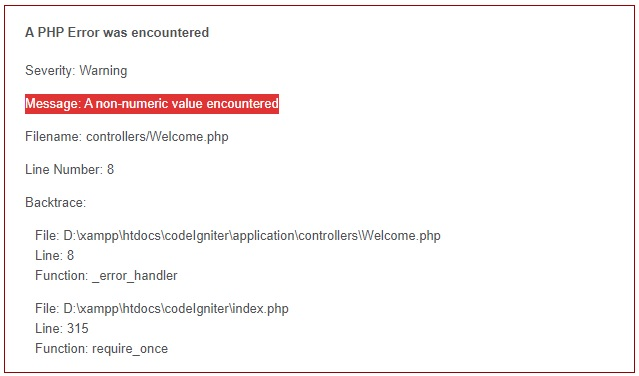# A non-numeric value encountered in PHP

Last updated 4 years, 2 months ago | 20962 views

Tags:- PHP# PHP | Warning: A non-numeric value encountered

This error occurs when you are trying to do a mathematical operation on an integer with a nun-numeric value or a string

Info: New E_WARNING and E_NOTICE errors have been introduced when invalid strings are coerced using operators expecting numbers (+ - * / ** % << >> | & ^) or their assignment equivalents. An E_NOTICE is emitted when the string begins with a numeric value but contains trailing non-numeric characters, and an E_WARNING is emitted when the string does not contain a numeric value.

You can solve the problem by just casting the thing into the number.

``````<?php
// casting the value
\$total = (int)\$value_1 + (int)\$value_2;
echo \$total;
?>``````

OR you can do some logic if you are taking value from the user, it is good practice to check it before doing any operation.

``````<?php
// Form value
\$value_1 = \$_POST['value1'];
\$value_2 = \$_POST['value2'];

// is_numeric function is use to change the value is numeric or not
if (is_numeric(\$value_1) && is_numeric(\$value_2)) {
\$total = \$value_1 + \$value_2;
echo \$total;
} else {
// do some error handling...
}
?>``````